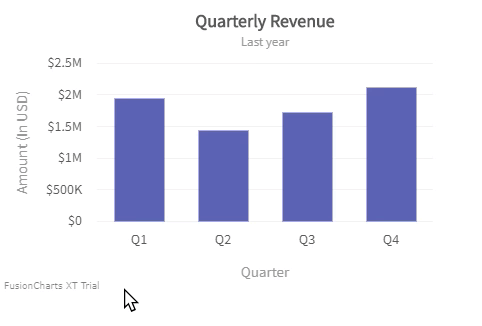# Data Plot

Data plot refers to the columns of the column chart, lines in a line chart, pie/doughnut slices in a pie/doughnut chart. You can enhance the way your data plot looks using colors, gradients and hover effects.

## Color individual data plots

You can specify a custom color for each data plot. Specify the hex code of the color using the `color` attribute within `data`under the `chart` object.

Refer to the code below:

``````{
"chart": {
...
},
"data": [
{
"label": "Jan",
"value": "420000"
}, {
"label": "Feb",
"value": "810000"
}, {
"label": "Mar",
"value": "720000"
}, {
"label": "Apr",
"value": "550000"
}, {
"label": "May",
"value": "910000",
"color": "#9b59b6" //Custom Color
}, {
"label": "Jun",
"value": "510000"
}, {
"label": "Jul",
"value": "680000"
}, {
"label": "Aug",
"value": "620000"
}, {
"label": "Sep",
"value": "610000"
}, {
"label": "Oct",
"value": "490000"
}, {
"label": "Nov",
"value": "900000",
"color": "#e44a00"  //Custom Color
}, {
"label": "Dec",
"value": "730000"
}
]
}``````

The chart will look like as shown below:

## Color different data plots

You can also specify a comma separated list of colors for use in different data series (or in case of single series charts, different data plots). The colors are selected from the list in a round-robin way for each data series.

Specify your custom palette for data plots by providing a list of hex colors using the `paletteColors` attribute. Refer to the code below:

``````{
"chart": {
"palettecolors": "5d62b5,29c3be,f2726f"
}
}``````

The chart will look like as shown below:

You can apply a global gradient color for an entire data plot. To do so follow the steps given below:

• `usePlotGradientColor` - Set this attribute to `1` to turn use the gradient effect.

• `plotGradientColor` - Specify the hex code of the gradient color.

Refer to the code below:

``````{
"chart": {
}
}``````

The chart will look like as shown below:

Apart from a basic gradient effect, you can also customize the gradient angle and fill ratio for a data plot. To customize the gradient properties, the following attributes are used:

• `plotFillAngle` - Set the fill angle for the gradient (for column, area and pie charts). Values can range from `0-360`.

• `plotFillRatio` - Specify the start and end of the gradient effect in a comma separated format. For example, a setting of "20, 40" would cause the gradient effect to start at 20% of width and end at 40% of the width. The color before gradient start would be set to the data plot color, and after the gradient end would get set to the gradient fill color.

• `plotFillAlpha` - Set the transparency of the gradient fill.

Refer to the code below:

``````{
"chart": {
"plotFillAngle": "0",
"plotFillAlpha": "60",
"plotFillRatio": "90,100"
}
}``````

The chart will look like as shown below:

## Add pattern fill to data plots

Instead of solid colors and gradients, you can also use patterns to fill data plots. You can control different pattern characteristics like type, color, angle, and opacity. In single series charts you can define patterns at a global or local level, in multi-series charts for each series. Defining patterns at local level overwrites global values for a particular data plot or series.

You can use pattern fills in the following charts: All 2D Column and Area charts, Pie2D, Doughnut2d, Multi-level Pie chart, Bubble, Funnel, and Pyramid charts. For 2D Combination charts only for column and area plot.

To use patterns in a chart set the `usePattern` attribute to `1`, refer to the code below:

``````{
"chart": {
"usePattern": "1"
...
}
}``````

To customize your pattern fill you can use the following attributes:

• `patternType` - Sets the type of pattern you want to use, the possible values for this attribute are: `circle`, `square`, and `line`. When you don't set this attribute the default pattern type used is `line`.
• `patternAngle` - Sets the angle of the pattern fill, it accepts values in degrees. The values can range from 0 to 360, the default value is 40 for lines and 0 for circle and square.
• `patternDensity` - Sets the density of pattern fill shapes increasing or decreasing the spacing between them, this attribute accepts values in pixels with a default value of 6.
• `patternSize` - Sets the size of pattern fill shapes, the behavior of this attribute depends on the type of pattern.
• For Line patterns it sets the thickness of lines, the default value is 2.
• For circle patterns it sets the diameter of circles, the default value is 4.
• For square patterns it sets the length of squares, the default value is 4.
• `color` - Sets the color of pattern shapes, it accepts values in hex color notation (#000000), by default patterns inherit the colors defined in `paletteColors`. you need to specify this attribute for each data plot in single series charts, and for each series in multi-series charts.
• `patternAlpha` - Sets the opacity of pattern shapes, it accepts values in % with a range of 0 to 100, the default value is 100.
• `patternBgColor` - Sets the data plot background color were the pattern fill is rendered, it accepts values in hex color notation (#000000), by default the background color is the same as the canvas or chart body. You need to specify this attribute for each data plot in single-series charts or for each series in multi-series charts.

Refer to the code below:

``````{
"chart": {
"usePattern": "1"
...
},
"data": [
{
"value": "190",
"patternType": "square",
"patternBgColor": "#1122ee",
"patternSize": 2,
"patternDensity": 8
}
]
}``````

The chart will look as show below:

## Show/hide plot border

Every data plot (column, area, or pie) has a border by default. Set the `showPlotBorder` to `0` to hide the plot border.

Refer to the code below:

``````{
"chart": {
"showPlotBorder": "0"
}
}``````

The chart will look like as shown below:

## Cosmetic options for plot border

FusionChart Version 3.71.0 extends the support to add data plot border cosmetics to all single-series and multi-series charts. The Data Plot Border Cosmetics consists of the following attributes:

• Use `plotBorderAlpha` attribute to specify the opacity for plot border at data plots and data-series levels.

• Use the `plotBorderColor` attribute to specify the color for plot border. Use the `inherit` value or set any hex code as the value to apply the respective plot color to the data plot and data-series.

• Use the `plotBorderThickness`attribute to specify the thickness for plot border at data plots and data-series levels.

• Use the `showPlotBorder` attribute to show or hide the plot border. When defined at data plot or data-series level it overwrites the value set at global level.

Refer to the code below:

``````{
"data": [{
"label": "Jan",
"showPlotBorder": "1",
"plotBorderThickness": "2",
"plotBorderColor": "#ff0000",
"plotBorderAlpha": "100",
"value": "420000"
}
}``````

The chart will look like as shown below:

## Top plot border of an area chart

In area charts, you can draw the borders of the data plots either only at the top of an area plot or on all the four sides of the area plot.

Take a look at the image with borders on all four sides of the are plot.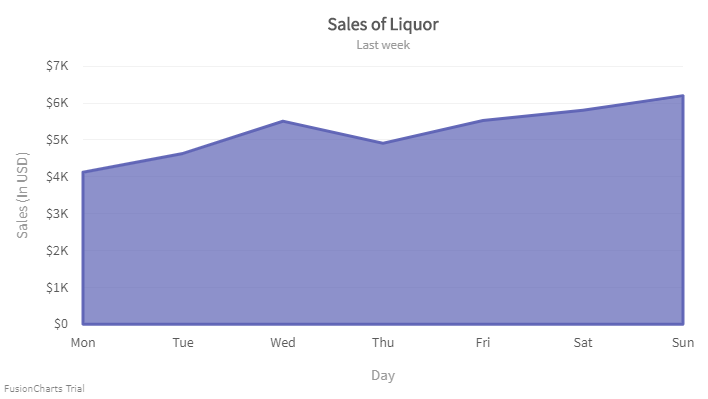Now, let's take a look an the image with the border only at the top of an area plot.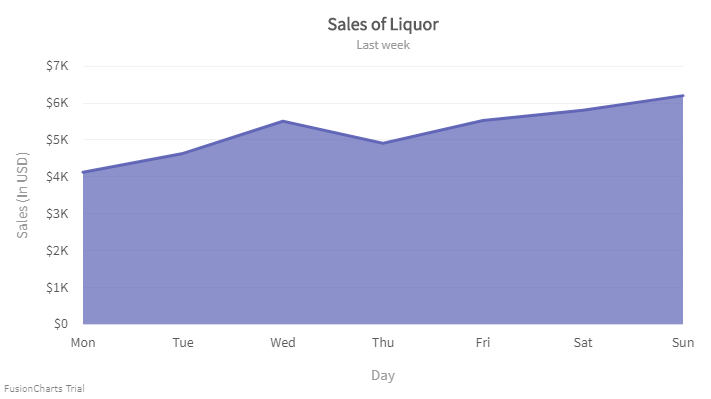To set the top border of the area plot follow the steps below:

• To set the top border of the area chart, set the value of `drawFullAreaBorder` attribute to `0`. This will work only if the `showPlotBorder` is set to `1`.

• Set the value of `drawFullAreaBorder` to `1` to draw the border around all four sides of an area chart.

• The default color of the border drawn with `drawFullAreaBorder` attribute would be black. It can be controlled by specifying the color in `plotbordercolor` attribute.

Refer to the code given below:

``````{
"chart": {
"showPlotBorder": "1",
"drawFullAreaBorder": "0"
}
}``````

You can also configure the top border of a particular data plot in an area chart with multiple data plots.

Take a look at the image given below: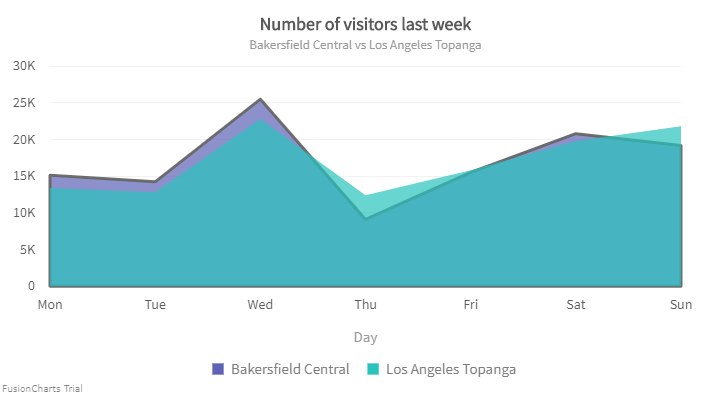To set the top border of a particular data plot in an area chart with multiple data plots, set the value of `drawFullAreaBorder` attribute to `0` under `dataset` object.

Refer to the code given below:

``````{
"chart": {
...
},
"categories": [
{
"category": [
...
]
}
],
"dataset": [
{
"seriesname": "Bakersfield Central",
"showPlotBorder": "1",
"drawFullAreaBorder": "0",
"plotBorderThickness": "3",
"data": [
...
]
}
]
}``````

The chart looks like as shown below:

## Inherit plot border color of an area chart

FusionCharts Suite allows you to set the border color of the area chart by inheriting the plot color.

A chart without inheriting the plot color looks like: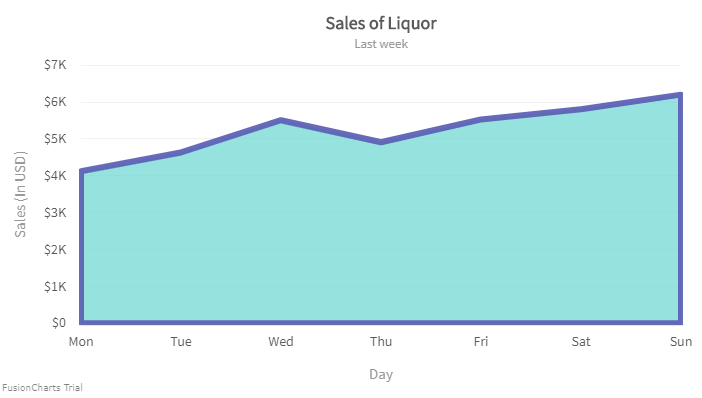To enable the plot border to inherit the color of an area plot, set the `inheritPlotBorderColor` attribute to `1`. By default, the value of the attribute is set to `0`.

Refer to the code given below:

``````{
"chart": {
"showPlotBorder": "1",
"inheritPlotBorderColor": "1"
}
}``````

The chart looks like as shown below:

Other `plotBorder` cosmetic attributes will work even if this attribute is set to `1`.

You can configure the `inheritPlotBorderColor` attribute for a particular data plot of an area chart with multiple data plots.

To inherit the color of the plot border from a particular data plot of an area chart with multiple data plots, set the value of `inheritPlotBorderColor` attribute to `1` under `dataset` object.

Refer to the code given below:

``````{
"chart": {
...
},
"categories": [
{
"category": [
...
]
}
],
"dataset": [
{
"seriesname": "Bakersfield Central",
"inheritPlotBorderColor": "1"
"data": [
...
]
}
]
}``````

The chart looks like as shown below:

## Dashed data plot border

You can set a dashed border for the data plot and can also customize dash-length and the gap between dashes. To do so, the following attributes are used:

• `plotBorderDashed` - Set this to `1` to make the border dashed.

• `plotBorderDashLen` - Set the length of each dash in plot-border (in pixels).

• `plotBorderDashGap` - Set the gap between two consecutive dashes in plot border (in pixels).

• `plotBorderThickness` - Set the thickness of the plot border.

• `plotBorderColor` - Set the hex color code of the plot border.

Refer to the code below:

``````{
"chart": {
"showPlotBorder": "1",
"plotBorderDashed": "1",
"plotBorderDashLen": "4",
"plotBorderDashGap": "4",
"plotBorderThickness": "1",
"plotBorderColor": "#000000"
}
}``````

The chart will look like as shown below:

The above attributes are used in the `chart` object which affects globally. You can also use dashed plot borders for specific data plots instead of the whole chart data plots. To do so, use the following attributes within `data` under the `chart` object:

• `dashed` - Set this to `1` to make the border dashed.

• `dashLen` - Set the length of each dash in plot-border (in pixels).

• `dashGap` - Set the gap between two consecutive dashes in plot border (in pixels).

The settings of these attributes will override the settings of the attributes under the `chart` object.

Refer to the code below:

``````{
"chart": {
...
},
"data": [{
"label": "Q1",
"value": "1950000",
"Dashed": "1",
"DashLen": "4",
"DashGap": "4",
}]
}``````

The chart will look like as shown below:

## Round edges

You can configure data plots with rounded edges in 2D Column or Bar charts. Set the `useRoundEdges` attribute to `1`.

Refer to the code below:

``````{
"chart": {
"useRoundEdges": "1"
}
}``````

The chart will look like as shown below:

## Plot hover effects

You can display hover effects for data plots to add an interactive element to the charts. To apply the hover effects at the `chart` level, the following attributes are used:

• `plotHoverEffect` - Set this attribute to `1` to enable hover effects for the data plots.

• `plotFillHoverColor` - Set the hover color for data plots in hex code format, e.g. `#00ffaa`.

• `plotFillHoverAlpha` - Set the transparency for hover color for data plots.

• `plotBorderHoverColor` - Set the hover border color in hex code format, e.g. `#00ffaa`.

• `plotBorderHoverAlpha` - Set the transparency of hover border for data plots.

• `plotBorderHoverThickness` - Set the hover border thickness (in pixels).

• `plotBorderHoverDashed` - Set this to `1` to make data plot borders appear dashed on hover.

• `plotBorderHoverDashLen` - Set the length of each dash for all data plots on hover(in pixels).

• `plotBorderHoverDashGap` - Set the gap between two consecutive dashes for all data plots on hover(in pixels).

Refer to the code below:

``````{
"chart": {
"plotHoverEffect": "1",
"plotFillHoverColor": "#00ffaa",
"plotBorderHoverThickness": "1",
"plotBorderHoverDashed": "1",
"plotBorderHoverDashLen": "6",
"plotBorderHoverDashGap": "2"
}
}``````

Shown below is a chart with hover effects enabled: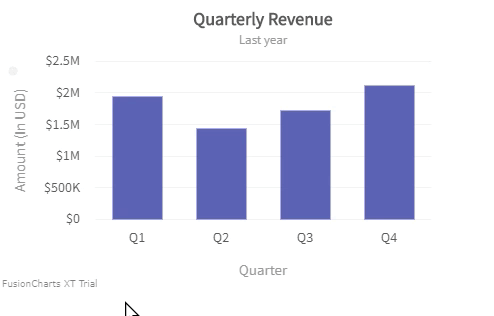You can also apply hover effects for individual data plots instead of applying them for all the data plots. To do so, you have to set the attributes within `data` for individual values. The attributes are:

• `hoverColor` - Set the hover color for data plots in hex code format, e.g. `#00ffaa`.

• `hoverAlpha` - Set the transparency for hover color for data plots.

• `borderHoverColor` - Set the hover border color in hex code format, e.g. `#00ffaa`.

• `borderHoverAlpha` - Set the transparency of hover border for data plots.

• `borderHoverThickness` - Set the hover border thickness (in pixels).

• `borderHoverDashed` - Set this to `1` to make data plot borders appear dashed on hover.

• `borderHoverDashLen` - Set the length of each dash for all data plots on hover (in pixels).

• `borderHoverDashGap` - Set the gap between two consecutive dashes for all data plots on hover(in pixels).

• `hoverGradientColor` - Set the gradient color of the data plot on hover (in pixels). For example, if you've specified individual colors and now you want hover color that ends in white, You need to specify it.

• `hoverRatio` - Set the ratio of the gradient color for data plots on hover.

• `hoverAngle` - Set the angle of the gradient color for data plots on hover.

All the above attributes will work only if `plotHoverEffect` is set to `1` under the chart object.

Refer to the code below:

``````{
"chart": {
"plotHoverEffect": "1"
},
"data": [
{
"label": "Q1",
"value": "1950000",
"HoverColor": "1",
"HoverAlpha": "1"
},
{
"label": "Q2",
"value": "1450000"
}
]
}``````

Shown below is a chart with hover effects enabled for an individual data plot: